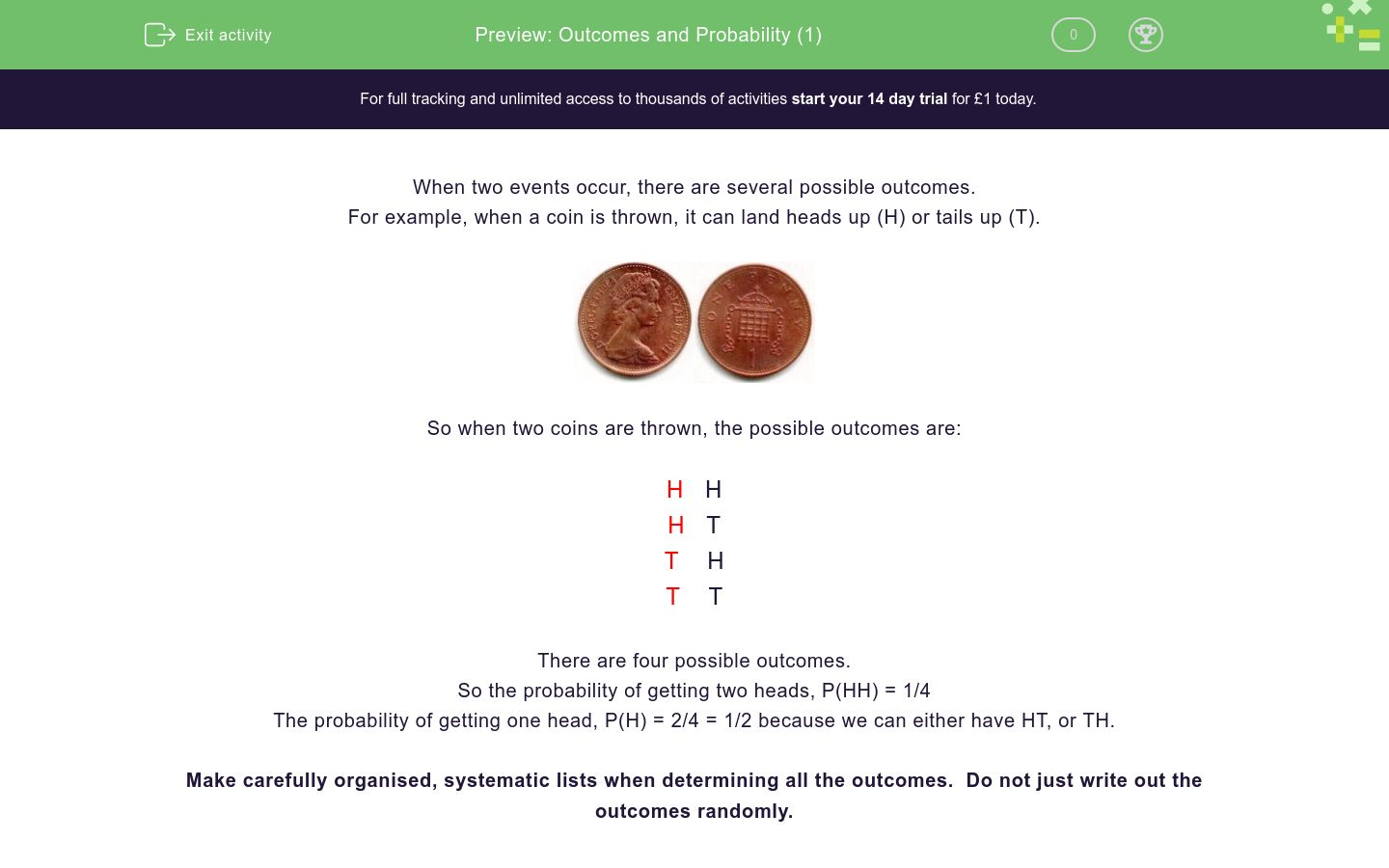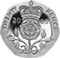# Outcomes and Probability (1)

In this worksheet, students determine the possible outcomes of two events.Key stage:  KS 3

Curriculum topic:   Probability

Curriculum subtopic:   Calculate Theoretical Probability

Difficulty level:### QUESTION 1 of 10

When two events occur, there are several possible outcomes.

For example, when a coin is thrown, it can land heads up (H) or tails up (T).So when two coins are thrown, the possible outcomes are:

H   H

H   T

T    H

T    T

There are four possible outcomes.

So the probability of getting two heads, P(HH) = 1/4

The probability of getting one head, P(H) = 2/4 = 1/2 because we can either have HT, or TH.

Make carefully organised, systematic lists when determining all the outcomes.  Do not just write out the outcomes randomly.

Two fair coins are thrown.Make a list of the possible outcomes on a piece of paper.

How many outcomes are there?

Two fair coins are thrown.Make a list of the possible outcomes on a piece of paper.

How many outcomes show two tails?

Two fair coins are thrown.Make a list of the possible outcomes on a piece of paper.

How many outcomes show one tail?

Two fair coins are thrown.Make a list of the possible outcomes on a piece of paper.

How many outcomes show no tails?

Two fair coins are thrown.Make a list of the possible outcomes on a piece of paper.

What is the probability of getting two tails?

One fair coin and one fair die are thrown.Make a list of the possible outcomes on a piece of paper.

H    1

H    2

H    3 ...

How many outcomes are there?

One fair coin and one fair die are thrown.Make a list of the possible outcomes on a piece of paper.

In how many outcomes is there a tail?

One fair coin and one fair die are thrown.Make a list of the possible outcomes on a piece of paper.

What is the probability of getting a tail?

(Write your answer with the / symbol, e.g. 2/3 and remember to reduce your fraction to its lowest terms)

One fair coin and one fair die are thrown.Make a list of the possible outcomes on a piece of paper.

In how many outcomes is there a tail and an even number?

One fair coin and one fair die are thrown.Make a list of the possible outcomes on a piece of paper.

What is the probability of getting a tail on the coin and an even number on the die?

(Write your answer with the / symbol, e.g. 2/3 and remember to reduce your fraction to its lowest terms)

• Question 1

Two fair coins are thrown.Make a list of the possible outcomes on a piece of paper.

How many outcomes are there?

4
• Question 2

Two fair coins are thrown.Make a list of the possible outcomes on a piece of paper.

How many outcomes show two tails?

1
• Question 3

Two fair coins are thrown.Make a list of the possible outcomes on a piece of paper.

How many outcomes show one tail?

2
• Question 4

Two fair coins are thrown.Make a list of the possible outcomes on a piece of paper.

How many outcomes show no tails?

1
• Question 5

Two fair coins are thrown.Make a list of the possible outcomes on a piece of paper.

What is the probability of getting two tails?

1/4
• Question 6

One fair coin and one fair die are thrown.Make a list of the possible outcomes on a piece of paper.

H    1

H    2

H    3 ...

How many outcomes are there?

12
• Question 7

One fair coin and one fair die are thrown.Make a list of the possible outcomes on a piece of paper.

In how many outcomes is there a tail?

6
• Question 8

One fair coin and one fair die are thrown.Make a list of the possible outcomes on a piece of paper.

What is the probability of getting a tail?

(Write your answer with the / symbol, e.g. 2/3 and remember to reduce your fraction to its lowest terms)

1/2
• Question 9

One fair coin and one fair die are thrown.Make a list of the possible outcomes on a piece of paper.

In how many outcomes is there a tail and an even number?

3
• Question 10

One fair coin and one fair die are thrown.Make a list of the possible outcomes on a piece of paper.

What is the probability of getting a tail on the coin and an even number on the die?

(Write your answer with the / symbol, e.g. 2/3 and remember to reduce your fraction to its lowest terms)

1/4
---- OR ----

Sign up for a £1 trial so you can track and measure your child's progress on this activity.

### What is EdPlace?

We're your National Curriculum aligned online education content provider helping each child succeed in English, maths and science from year 1 to GCSE. With an EdPlace account you’ll be able to track and measure progress, helping each child achieve their best. We build confidence and attainment by personalising each child’s learning at a level that suits them.

Get started